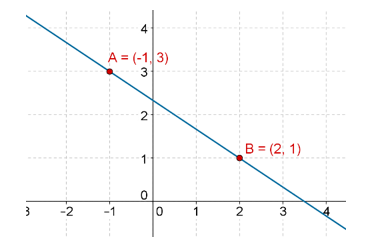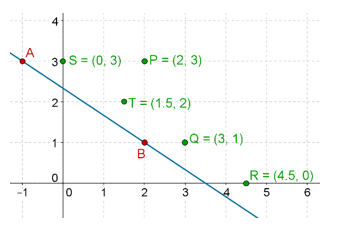# Linear Inequalities in Two Variables

Go back to  'Linear-Equations'

A linear inequation in two variables is formed when one expression is put as greater than or less than another expression, and two variables are involved. Here are some examples of linear inequations in two variables:

$\begin{array}{l}2x< 3y + 2\\7x - 2y > 8\\3x + 4y + 3 \le 2y - 5\\y + x \ge 0\end{array}$

Once again, as shown above, we can have strict inequalities, in which the two sides of the inequation cannot be equal to each other, or we can have non-strict inequalities, in which the two sides of the inequation can also be equal.

Consider the following inequation:

$2x +3y > 7$

When we talk about finding the solution to this inequation, we are talking about all those pairs of values of x and y for which this inequation is satisfied. This means,for example, that $$x = 4,\;y = 3$$ is one possible solution to this particular inequation, but $$x = 0,\;y = 0$$ is not, because when you substitute x and y equal to 0 on the LHS of the inequation, it turns out to be less than 7.

We saw that for any linear equation in two variables, there are infinitely many solutions. It might be obvious to you now that for any linear inequation as well, we will have infinitely many solutions. All these solutions will constitute the solutionset of the linear inequation. Therefore, when we say something like: solve this linear inequation, we are actually saying: determine the solution set for this linear inequation.

What will be the solution set for the inequation above? And more importantly, how do we represent it graphically? The solution set for a linear equation corresponded graphically to a straight line. What will the solution set of a linear inequation correspond to?

To answer these questions, let us plot the straight line corresponding to the linear equation $$2x + 3y = 7$$. We determine any two points(solutions) for this equation:

$\begin{array}{l}x = - 1\;\; \Rightarrow \;\;\;y = 3\\x = 2 \;\;\; \Rightarrow \;\;\;y =1\end{array}$

Thus, two possible points on the graph can be taken as $$A\left( { - 1,\;3}\right),\,\,B\left( {2,\;1} \right)$$. Now, we plot the graph:Next, we determine some specific solutions for the linear inequation

$2x + 3y > 7$

That is, we find out, say, five points whose coordinates will satisfy this linear inequation. For example,

$\begin{array}{l}P\left({2,3} \right),\,\,\,Q\left( {3,1} \right),\,\,\,R\left( {4.5,0}\right),\,\,\\S\left( {0,3} \right),\,\,\,T\left( {1.5,2} \right)\end{array}$

Now, let’s plot these five points on the same graph as the one we obtained for the linear equation. We will obtain a plot like the one below:We notice an interesting pattern: all the five points (corresponding to the five solutions) lie above the line. Is this a coincidence? Or is there something more involved? In fact, if you take any point which lies above the line, its coordinates, say $$\left( {{x_0},\;{y_0}} \right),$$ will satisfy the  inequation:

$2{x_0} + 3{y_0} >7$

This means that the solution set for the inequation consists of all points lying above the line. What about the solution set for the inequation

$2x + 3y < 7$

You might have guessed that the solution set for this inequation consists of all points lying below the line. Let us summarize these facts:

### For points lying above the line:

$2x + 3y > 7$

### For points lying on the line:

$2x + 3y = 7$

### For points lying below the line:

$2x + 3y < 7$

Any line will divide the plane in which it lies into two half-planes. Thus, we see that the solution sets of linear inequations correspond to half-planes, while the solution sets of linear equations corresponds to lines. These observations are extremely important and it is imperative that you understand them properly.

Learn math from the experts and clarify doubts instantly

• Instant doubt clearing (live one on one)
• Learn from India’s best math teachers
• Completely personalized curriculum